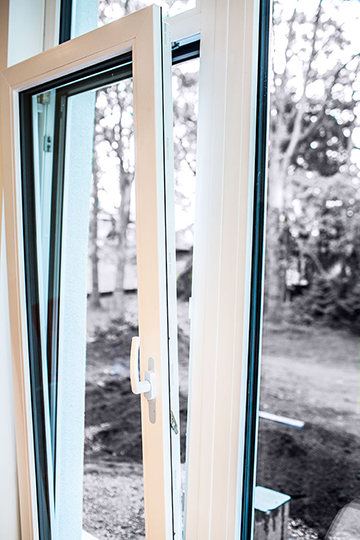# How to find entropy production of opening the windows in the winter?

Every time you open the windows in the winter (to bring in healthy and fresh air), room's warm air goes outside into the atmosphere.

How to find entropy production of opening the windows in the winter?

The room's volume is denoted by $V_r$ and its temperature by $T_r$, and atmospheric volume is denoted by $V_a$ and its temperature by $T_a$.• That surely depends on the rate of heat transfer, which itself depends on lots of other factors... – lemon Jun 17 '16 at 11:58
• See the equation here web.mit.edu/16.unified/www/SPRING/propulsion/notes/node40.html for "Calculation of Entropy Change: Heat transfer between two heat reservoirs" let me know if you have any questions – pentane Jun 17 '16 at 15:06
• the complication is that it is not just heat transfer, it is also mass transfer, as the densities of air are initially different – Wolphram jonny Jun 17 '16 at 15:10
• @pentane The mean problem is that Atmosphere is Dynamic because it changes. – Achmed Jun 18 '16 at 13:43
• Which system is supposed to be looked at in terms of entropy change, the room or the atmosphere? – jjack Dec 23 '17 at 16:44

Note: We need one property of the room's air for example mass or pressure of it.

From "THERMODYNAMICS An Engineering Approach, Fifth Edition, by YUNUS A. CENGEL and MICHAEL A. BOLES":

Irreversibilities such as friction, mixing, chemical reactions, heat transfer through a finite temperature difference, unrestrained expansion, nonquasiequilibrium compression or expansion always generate entropy, and anything that generates entropy always destroys exergy. The exergy destroyed is proportional to the entropy generated: $$X_{\textrm{destroyed}}=T_0S_{\textrm{gen}}\ge 0$$

So, assuming whole of the room's air is replaced with outside air, current question is reduced to:

Calculate the exergy of a room that contains air by the volume of $V_r$ and temperature of $T_r$ while the environment is at temperature of $T_a$ and pressure of $P_a$ (I assume we know the pressure, if not we can calculate it by using ideal gas law $P_a=\frac{RT_a}{V_a}$)

Because according to equation above we have: $S_{\textrm{gen}}=\frac{X_{\textrm{destroyed}}}{T_a}$ and if we assume that no useful work is done, then we have $X_{\textrm{destroyed}}=X_{\textrm total}$ (I.e. whole of exergy is destroyed)

Now let's calculate the exergy of the room before opening the window:

From the same book we have: $$X=m\left[(u-u_0)+P_0(v-v_0)-T_0(s-s_0)+\frac{\mathscr V^2}2+gz\right]$$

So that:

$X$ is exergy of closed$^1$ system

$m$ is the mass of the system

$u$ is the internal energy of unit mass

$P$ is the pressure

$T$ is the temperature

$s$ is the entropy of unit mass

$\mathscr V$ is the speed of the system (Whole air of the room)

$z$ is the height of the center of mass of the system

The subscript of $0$ refers to the environment state.

The room is fixed. So, we have: $\mathscr V=0$

Also we can ignore the potential energy of the air, i.e. $gz=0$

So, we will have: $$X=m\left[(u-u_a)+P_a(v-v_a)-T_a(s-s_a)\right]$$

As we have two independent intensive properties of inside and outside air (we know temperature and pressure of them), we can determine all quantities of latter equation.

Once we found $X$, we can say $S_{\textrm {gen}}=\frac X{T_a}$ so that $S_{\textrm {gen}}$ is entropy generation.

$^1$ Our system is a closed system because we want to calculate the exergy of the system before opening the window.

• Why don't you answer the question without involving exergy? Seems to be more optimal with respect to gaining an understanding. – jjack Dec 23 '17 at 15:07

Step 1) Find a model for the gas in the room. We might as well take an ideal gas for this demonstration; I think this is generally an alright model for the air since it's mostly inert nitrogen.

Step 2) Apply the first law of thermodynamics, ignore the work done in opening the window. The first law now states

$$\Delta S = \int \limits_{T_0}^{T_f} \frac{dE}{T}$$

because the room has rigid walls and thus $dV = 0$.

Step 3) The average energy in the room associated with an ideal gas as a function of temperature is $E(T)=c_v n R T$.

Step 4) Plug in $dE(T) = c_v n R\: dT$ and integrate:

$$\Delta S = c_v n R \ln\left(\frac{T_f}{T_0}\right)$$